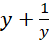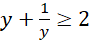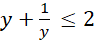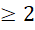# CAT 2019 Question Paper | Quants Slot 1

###### CAT Previous Year Paper | CAT Quants Questions | Question 17

Here is a slightly tough question in CAT 2019. It combines the topics of trigonometry and algebra. It is very important to have done a solid CAT Preparation to be sure about the concepts and trigonometry formula to even attempt this question. Once you are clear with the concepts & formulas, it would be prudent to practice them from CAT previous year paper.

Question 17 : The number of the real roots of the equation 2cos(x(x + 1)) = 2x + 2-x is

1. 0
2. Infinite
3. 1
4. 2

## Best CAT Coaching in Chennai

#### CAT Coaching in Chennai - CAT 2022Limited Seats Available - Register Now!

2cos (x(x + 1)) = 2x + 2-x
Consider RHS, 2x + 2-x
This is of the form,We know thatwhen y is positive andwhen y is negative
Here, since we are dealing with 2x,is considered
So, 2cos (x (x + 1))Substitute x = 0, 2x + 2-x = 20 + 20 = 2
2cos (x (x + 1)) = 2cos (0(0 + 1)) = 2cos (0) = 2 x = 0 is a valid solution
Any value other than this wont work.
Therefore, only x = 0 works and there is only one real solution

The question is "The number of the real roots of the equation 2cos(x(x + 1)) = 2x + 2-x is "

##### Hence, the answer is 1

Choice C is the correct answer.

###### CAT Coaching in ChennaiCAT 2023

Classroom Batches Starting Now! @Gopalapuram

###### Best CAT Coaching in Chennai Introductory offer of 5000/-

Attend a Demo Class

##### Where is 2IIM located?

2IIM Online CAT Coaching
A Fermat Education Initiative,
58/16, Indira Gandhi Street,
Kaveri Rangan Nagar, Saligramam, Chennai 600 093

##### How to reach 2IIM?

Mobile: (91) 99626 48484 / 94459 38484
WhatsApp: WhatsApp Now
Email: info@2iim.com